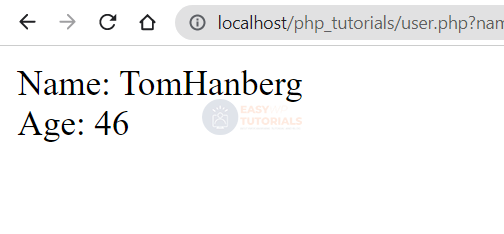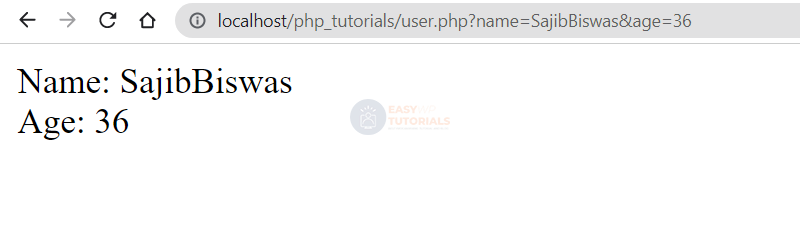# Getting data from a query string in PHP

The simplest way to pass data to the server to a PHP application from the outside is to pass data through the query string.

The query string represents a set of parameters that are placed after the question mark in the address. Each parameter defines a name and a value. For example, in the address:

http://localhost/user.php?name=TomHanberg&age=46

The part ?name=Tom&age=36represents a query string that has two parameters name and age. Each parameter has a name and a value, separated by an equal sign. The parameter name has the value “Tom” and the parameter has the age value 36. The parameters are separated from each other by an ampersand.

For example, let’s define the following user.php script with the following content:

```<?php
\$name = "not defined";
\$age = "not defined";
if(isset(\$_GET["name"])){

\$name = \$_GET["name"];
}
if(isset(\$_GET["age"])){

\$age = \$_GET["age"];
}
echo "Name: \$name <br> Age: \$age";
?>
```

When we enter an address into the address bar of the browser and click on submit, a GET request is sent to the server. PHP defines by default a global associative array \$_GET that stores all values ​​passed in GET requests. Using the keys of the transmitted data, we can get the transmitted values ​​from the \$_GET array.

When sending a query string, the keys in this array will be the names of the parameters, and the values ​​will be the values ​​of the parameters.

For example, a parameter is passed in a query string name=Tom. Accordingly, to get the value of the name parameter from the request, we access the corresponding key:

```\$name = \$_GET["name"];  // Tom
```

However, it should be borne in mind that the address bar will not necessarily use the query string or this particular parameter. Therefore, before receiving the parameter value, we first look to see if such a parameter was passed at all:

```if(isset(\$_GET["name"])){
```

Now let’s turn to this script, for example, like this http://localhost/user.php?name=Tom&age=36 :If we do not pass the value of any parameter, then the corresponding variable will use the default value: